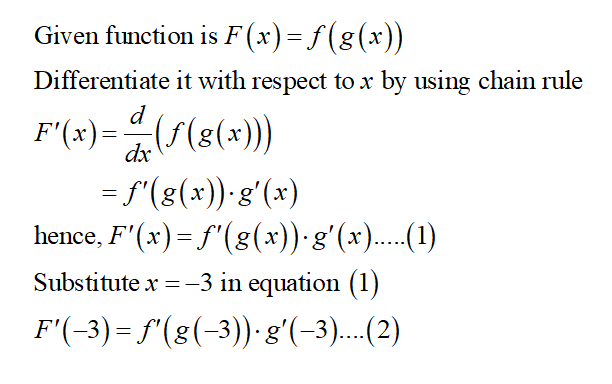# If F(x) = f(g(x)), where f(−4) = 5, f '(−4) = 6, f '(−3) = 5, g(−3) = −4, andg'(−3) = 8, find F '(−3). question one:  F '(−3) =

Question
19 views

If F(x) = f(g(x)), where f(−4) = 5, f '(−4) = 6, f '(−3) = 5, g(−3) = −4, and

g'(−3) = 8, find F '(−3).

question one:  F '(−3) =

check_circle

star
star
star
star
star
1 Rating
Step 1...

### Want to see the full answer?

See Solution

#### Want to see this answer and more?

Solutions are written by subject experts who are available 24/7. Questions are typically answered within 1 hour.*

See Solution
*Response times may vary by subject and question.
Tagged in